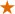#### File Exchange > Data Analysis >    Gaussian Mixture ModelsAuthor:
OriginLab Technical Support
7/23/2018
Last Update:
4/25/2023
514
Total Ratings:
4
File Size:
508 KB
Average Rating:File Name:
FitGMM.opx
File Version:
1.10
Minimum Versions:
Free
Type:
App
Summary:

Fit Gaussian mixture models and cluster test data.

Description:

PURPOSE
This app can be used to fit Gaussian mixture model using EM method, estimate parameters in the distribution, calculate probability density function and classify test data by posterior probability. It can also find the best model by BIC. It can create several graphs including histogram, probability density function plot, cumulative distribution function plot, Q-Q plot, contour plot and confidence region plot according to the number of variables for input data.

INSTALLATION
Download FitGMM.opx file, and then drag-and-drop onto the Origin workspace. An icon will appear in the Apps Gallery window.
NOTE: This tool requires OriginPro.

OPERATION

1. Make a worksheet for input data active. Click the Gaussian Mixture Models icon in the Apps Gallery window.
2. In the opened dialog, select columns from the worksheet as Variables in Input tab. Input data can be one variable, two variables, three variables or more than three variables. If group is known, choose it as Initial Group to estimate initial values for the distribution, Initial Group is an optional input. If you want to predict test data, check Predict Test Data edit box, and select columns for Test Data. Note that number of columns for test data must be same as that for Variables.
3. In the Settings tab, choose the type to specify number of components for the mixture distribution: by A Fixed Number or BIC. For BIC type, it will find the optimal number of components whose BIC is the smallest. Choose Covariance Matrix type: Groupwise or Pooled, the former option will use different covariance matrices for different group levels while the latter option will use the same. Set an integer value for Maximum Number of Iterations and a value to Tolerance for log(Likelihood).
4. In the Quantities tab, choose which quantities to compute. Quantities options include Mixing ProportionMean and Covariance Matrix in Fit Parameters branch, log Likelihood and BIC in Fit Statistics branch, Probability DensityPosterior Probability and Predicted Group for both training data and test data in Fitted Result branch.
5. In the Plots tab, following plots are available: Histogram (one variable), Probability Density Function Plot (one or two variables), Cumulative Distribution Function Plot (one variable), Q-Q Plot (one variable), Contour Plot (two variables) and Confidence Region Plot (two or three variables). Grid Size in Each Dimension can be defined for contour or surface plots, and Confidence Level (%) option is available for confidence region plot.
6. Click OK button, a report sheet, a report data sheet and a plot data sheet will be created.

Sample OPJU File
This app provides a sample OPJU file.  Right click on the Gaussian Mixture Models icon in the Apps Gallery window, and choose Show Samples Folder from the short-cut menu. A folder will open. Drag-and-drop the project file FitGMMSample.opju from the folder onto Origin. The Notes window in the project shows detailed steps.
Note: If you wish to save the OPJU after changing, it is recommended that you save to a different folder location (e.g. User Files Folder).

NOTES

1. If a row in input data contains one or more missing values, the entire row will be excluded from the analysis.
2. Number of rows for input data must be greater than number of parameters.
3. This tool can be used to process the score result in Principal Component Analysis.

 11/24/2022 OriginLab Hi Victorfeynman, Thanks for the suggestion. We have submitted a jira(APPS-1233) to follow up this request. Thanks, Shirley 11/23/2022 Victorfeynman I am currently using GMM to cluster my data. I need a plot of BIC against a number of components. The program only determines the optimal states of the data, how do I get this plot, showing BIC values beyond the optimal number. Thank you.11/21/2022 15737342116 No04/07/2021 Zeng Kai